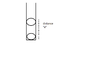# Plastic Balls in a Test Tube with Mass

PeachBanana

## Homework Statement

One charged plastic ball is vertically above another in a test tube as shown. The balls are in equilibrium a distance "d" apart. If the mass of the upper ball is reduced by a factor of four, the distance between the balls in the test tube would become :

1) 4d
2) 8d
3) 2d
4) square root of 2d

?

## The Attempt at a Solution

How are charge and mass related? That's what I can't understand.

#### Attachments

•physics.png
4.3 KB · Views: 494

Homework Helper
Charge decides the repulsive force and mass decides the gravitational attractive force. In equilibrium position they are equal amid opposite.

PeachBanana
Ok. I'm thinking along the lines of

F initial = G m1m2 / r^2

F final = G 1/4m1m2/ r^2

But I need to put those in terms of "r" and compare, right?

Homework Helper
By changing mass of tthe plastic ball, the weight mg changes. Now compare the two weights with the force of repulsion.

Last edited:
PeachBanana
Ok. What I just did was

Gm1m2
--------
1/4 Gm1m2

to get a 4:1 ratio. Yet I didn't use anything with "R."

Homework Helper
When mass decreases, the weight decreases. To balance this the charges must move farther. What is the formula for the force of repulsion?

PeachBanana
F = kq1q2/r^2 ?

Homework Helper
Curret. Now if the initial distance for equilibrium is d for mg , what is new distance to balance mg/4?

PeachBanana
I'm thinking:

d initial = mg
--------------
d final 1/4 mg

solving for d initial I get:

d initial = 4d final

But there's a square involved so shouldn't the answer be "2d?"

Homework Helper
Yes.